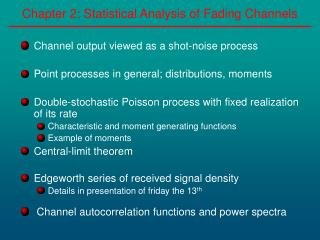Download PresentationChapter 2: Statistical Analysis of Fading Channels

# Chapter 2: Statistical Analysis of Fading Channels

Download Presentation## Chapter 2: Statistical Analysis of Fading Channels

- - - - - - - - - - - - - - - - - - - - - - - - - - - E N D - - - - - - - - - - - - - - - - - - - - - - - - - - -
##### Presentation Transcript

1. Chapter 2: Statistical Analysis of Fading Channels • Channel output viewed as a shot-noise process • Point processes in general; distributions, moments • Double-stochastic Poisson process with fixed realization of its rate • Characteristic and moment generating functions • Example of moments • Central-limit theorem • Edgeworth series of received signal density • Details in presentation of friday the 13th • Channel autocorrelation functions and power spectra

2. Chapter 2: Shot-Noise Channel Simulations • Channel Simulations Experimental Data (Pahlavan p. 52)

3. Chapter 2: Shot-Noise Channel Model

4. Chapter 2: Shot-Noise Effect ti ti • Channel viewed as a shot-noise effect [Rice 1944] Linear system Counting process Response Shot-Noise Process: Superposition of i.i.d. impulse responses occuring at times obeying a counting process, N(t).

5. Chapter 2: Shot-Noise Effect • Measured power delay profile

6. Chapter 2: Shot-Noise Definition • Shot noise processess and Campbell’s theorem

7. Chapter 2: Wireless Fading Channels as a Shot-Noise • Shot-Noise Representation of Wireless Fading Channel

8. Chapter 2: Shot-Noise Assumption • Counting process N(t): Doubly-Stochastic Poisson Process with random rate

9. Chapter 2: Joint Characteristic Function • Conditional Joint Characteristic Functional of y(t)

10. Chapter 2: Joint Moment Generating Function • Conditional moment generating function of y(t) • Conditional mean and variance of y(t)

11. Chapter 2: Joint Characteristic Function • Conditional Joint Characteristic Functional of yl(t)

12. Chapter 2: Joint Moment Generating Function • Conditional moment generating function of yl(t) • Conditional mean and variance of yl(t)

13. Chapter 2: Correlation and Covariance • Conditional correlation and covariance of yl(t)

14. Chapter 2: Central-Limit Theorem • Central Limit Theorem • yc(t)is a multi-dimensional zero-mean Gaussian process with covariance function identified

15. Chapter 2: Edgeworth Series Expansion • Channel density through Edgeworth’s series expansion • First term: Multidimensional Gaussian • Remaining terms: deviation from Gaussian density

16. Chapter 2: Edgeworth Series Simulation • Channel density through Edgeworth’s series expansion • Constant-rate, quasi-static channel, narrow-band transmitted signal

17. Chapter 2: Edgeworth Series vs Gaussianity • Channel density through Edgeworth’s series expansion • Parameters influencing the density and variance of received signal depend on • Propagation environment Transmitted signal • l(t) l(t) TsTs(signal. interv.) • s (var. I(t),Q(t)) K • rs

18. Chapter 2: Channel Autocorrelation Functions Fc(t ) Power Delay Profile |Fc(Df)| t Tm Ft Bc Df Dt=0 Power Delay Spectrum Fc( Dt;t ) Ft FDt |Fc(Dt;Df)| Dt=0 Scattering Function Df WSSUS Channel Sc( l;t ) Dt Sc(l; Df) Ft FDt Df=0 Df=0 Sc(l;t) |Fc(Dt)| Sc( l ) t Doppler Power Spectrum Tc Dt FDt l Bd l

19. Chapter 2: Channel Autocorrelations and Power-Spectra • Consider a Wide-Sense Stationary Uncorrelated Scattering (WSSUS) channel with moving scatters • Non-Homogeneous Poisson rate: l(t) • ri(t,t) = ri(t): quasi-static channel • pf(f)=1/2p , pq(q)=1/2p

20. Chapter 2: Channel Autocorrelations and Power-Spectra • Time-spreading: Multipath characteristics of channel

21. Chapter 2: Channel Autocorrelations Power-Spectra • Time-spreading: Multipath characteristics of channel

22. Chapter 2: Channel Autocorrelations and Power-Spectra • Time-spreading: Multipath characteristics of channel • Autocorrelation in Frequency Domain, (space-frequency, space-time)

23. Chapter 2: Channel Autocorrelations and Power-Spectra Double Fourrier transform • Time variations of channel: Frequency-spreading:

24. Chapter 2: Channel Autocorrelations and Power-Spectra • Time variations of channel: Frequency-spreading

25. Chapter 2: Channel Autocorrelations and Power-Spectra • Time variations of channel: Frequency-spreading

26. Chapter 2: Shot-Noise Simulations • Temporal simulations of received signal

27. Chapter 2: References • K.S. Miller. Multidimentional Gaussian Distributions. John Wiley&Sons, 1964. • S. Karlin. A first course in Stochastic Processes. Academic Press, New York 1969. • A. Papoulis. Probability, Random Variables and Stochastic Processes. McGraw Hill, 1984. • D.L. Snyder, M.I. Miller. Random Point Processes in Time and Space. Springer Verlag, 1991. • E. Parzen. Stochastic Processes. SIAM, Classics in Applied Mathematics, 1999. • P.L. Rice. Mathematical Analysis of random noise. Bell Systems Technical Journal, 24:46-156, 1944. • W.F. McGee. Complex Gaussian noise moments. IEEE Transactions on Information Theory, 17:151-157, 1971.

28. Chapter 2: References • R. Ganesh, K. Pahlavan. On arrival of paths in fading multipath indoor radio channels. Electronics Letters, 25(12):763-765, 1989. • C.D. Charalambous, N. Menemenlis, O.H. Karbanov, D. Makrakis. Statistical analysis of multipath fading channels using shot-noise analysis: An introduction. ICC-2001 International Conference on Communications, 7:2246-2250, June 2001. • C.D. Charalambous, N. Menemenlis. Statistical analysis of the received signal over fading channels via generalization of shot-noise. ICC-2001 International Conference on Communications, 4:1101-1015, June 2001. • N. Menemenlis, C.D. Charalambous. An Edgeworth series expansion for multipath fading channel densities. Proceedings of 41stIEEE Conference on Decision and Control, to appear, Las Vegas, NV, December 2002.